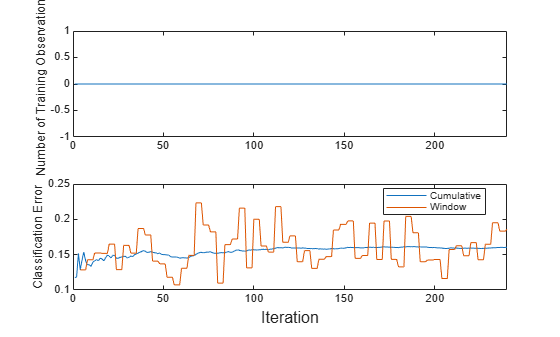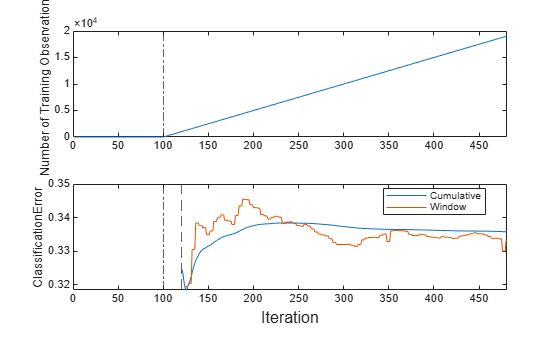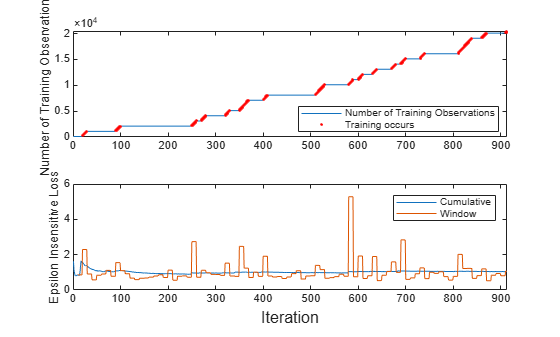# updateMetrics

Update performance metrics in kernel incremental learning model given new data

Since R2022a

## Syntax

``Mdl = updateMetrics(Mdl,X,Y)``
``Mdl = updateMetrics(Mdl,X,Y,Weights=weights)``

## Description

Given streaming data, `updateMetrics` measures the performance of a configured incremental learning model for kernel regression (`incrementalRegressionKernel` object) or binary kernel classification (`incrementalClassificationKernel` object). `updateMetrics` stores the performance metrics in the output model.

`updateMetrics` allows for flexible incremental learning. After you call the function to update model performance metrics on an incoming chunk of data, you can perform other actions before you train the model to the data. For example, you can decide whether you need to train the model based on its performance on a chunk of data. Alternatively, you can both update model performance metrics and train the model on the data as it arrives, in one call, by using the `updateMetricsAndFit` function.

To measure the model performance on a specified batch of data, call `loss` instead.

example

````Mdl = updateMetrics(Mdl,X,Y)` returns an incremental learning model `Mdl`, which is the input incremental learning model `Mdl` modified to contain the model performance metrics on the incoming predictor and response data, `X` and `Y` respectively.When the input model is warm (`Mdl.IsWarm` is `true`), `updateMetrics` overwrites previously computed metrics, stored in the `Metrics` property, with the new values. Otherwise, `updateMetrics` stores `NaN` values in `Metrics` instead.The input and output models have the same data type.```

example

````Mdl = updateMetrics(Mdl,X,Y,Weights=weights)` also sets observation weights.```

## Examples

collapse all

Train a kernel model for binary classification by using `fitckernel`, convert it to an incremental learner, and then track its performance to streaming data.

Load the human activity data set. Randomly shuffle the data.

```load humanactivity rng(1) % For reproducibility n = numel(actid); idx = randsample(n,n); X = feat(idx,:); Y = actid(idx);```

For details on the data set, enter `Description` at the command line.

Responses can be one of five classes: Sitting, Standing, Walking, Running, or Dancing. Dichotomize the response by identifying whether the subject is moving (`actid` > 2).

`Y = Y > 2;`

Train Kernel Model for Binary Classification

Fit a kernel model for binary classification to a random sample of half the data.

```idxtt = randsample([true false],n,true); TTMdl = fitckernel(X(idxtt,:),Y(idxtt))```
```TTMdl = ClassificationKernel ResponseName: 'Y' ClassNames: [0 1] Learner: 'svm' NumExpansionDimensions: 2048 KernelScale: 1 Lambda: 8.2967e-05 BoxConstraint: 1 ```

`TTMdl` is a `ClassificationKernel` model object representing a traditionally trained kernel model for binary classification.

Convert Trained Model

Convert the traditionally trained classification model to a model for incremental learning.

`IncrementalMdl = incrementalLearner(TTMdl)`
```IncrementalMdl = incrementalClassificationKernel IsWarm: 1 Metrics: [1x2 table] ClassNames: [0 1] ScoreTransform: 'none' NumExpansionDimensions: 2048 KernelScale: 1 ```

`IncrementalMdl` is an `incrementalClassificationKernel` model. The model display shows that the model is warm (`IsWarm` is `1`). Therefore, `updateMetrics` can track model performance metrics given data.

Track Performance Metrics

Track the model performance on the rest of the data by using the `updateMetrics` function. Simulate a data stream by processing 50 observations at a time. At each iteration:

1. Call `updateMetrics` to update the cumulative and window classification error of the model given the incoming chunk of observations. Overwrite the previous incremental model to update the losses in the `Metrics` property. Note that the function does not fit the model to the chunk of data—the chunk is "new" data for the model.

2. Store the classification error and number of training observations.

```% Preallocation idxil = ~idxtt; nil = sum(idxil); numObsPerChunk = 50; nchunk = floor(nil/numObsPerChunk); ce = array2table(zeros(nchunk,2),VariableNames=["Cumulative","Window"]); numtrainobs = [zeros(nchunk,1)]; Xil = X(idxil,:); Yil = Y(idxil); % Incremental fitting for j = 1:nchunk ibegin = min(nil,numObsPerChunk*(j-1) + 1); iend = min(nil,numObsPerChunk*j); idx = ibegin:iend; IncrementalMdl = updateMetrics(IncrementalMdl,Xil(idx,:),Yil(idx)); ce{j,:} = IncrementalMdl.Metrics{"ClassificationError",:}; numtrainobs(j) = IncrementalMdl.NumTrainingObservations; end```

`IncrementalMdl` is an `incrementalClassificationKernel` model object that has tracked the model performance to observations in the data stream.

Plot a trace plot of the number of training observations and the performance metrics on separate tiles.

```t = tiledlayout(2,1); nexttile plot(numtrainobs) ylabel("Number of Training Observations") xlim([0 nchunk]) nexttile plot(ce.Variables) xlim([0 nchunk]) ylabel("Classification Error") legend(ce.Properties.VariableNames,Location="best") xlabel(t,"Iteration")```The cumulative loss is stable, whereas the window loss jumps. The number of training observations does not change because `updateMetrics` does not fit the model to the data.

Configure incremental learning options for an `incrementalClassificationKernel` model object when you call the `incrementalClassificationKernel` function. Track the model performance on streaming data, and fit the model to the data. Specify observation weights when you call incremental learning functions.

Create an incremental kernel model for binary classification. Specify an estimation period of 5000 observations and the stochastic gradient descent (SGD) solver.

`Mdl = incrementalClassificationKernel(EstimationPeriod=5000,Solver="sgd")`
```Mdl = incrementalClassificationKernel IsWarm: 0 Metrics: [1x2 table] ClassNames: [1x0 double] ScoreTransform: 'none' NumExpansionDimensions: 0 KernelScale: 1 ```

`Mdl` is an `incrementalClassificationKernel` model. All its properties are read-only.

The model display shows that the model is not warm (`IsWarm` is `0`). Determine the size of the metrics warm-up period by displaying model properties.

`mwp = Mdl.MetricsWarmupPeriod`
```mwp = 1000 ```

Determine the number of observations that incremental fitting functions, such as `fit`, must process before measuring the performance of the model.

`numObsBeforeMetrics = Mdl.MetricsWarmupPeriod + Mdl.EstimationPeriod`
```numObsBeforeMetrics = 6000 ```

Load the human activity data set. Randomly shuffle the data.

```load humanactivity n = numel(actid); rng(1) % For reproducibility idx = randsample(n,n); X = feat(idx,:); Y = actid(idx);```

For details on the data set, enter `Description` at the command line.

Responses can be one of five classes: Sitting, Standing, Walking, Running, or Dancing. Dichotomize the response by identifying whether the subject is moving (`actid` > 2).

`Y = Y > 2;`

Suppose that the data collected when the subject was not moving (`Y` = `false`) has double the quality than when the subject was moving. Create a weight variable that attributes 2 to observations collected from a still subject, and 1 to a moving subject.

`W = ones(n,1) + ~Y;`

Perform incremental learning. At each iteration:

• Simulate a data stream by processing a chunk of 50 observations.

• Measure model performance metrics on the incoming chunk using `updateMetrics`. Specify the corresponding observation weights and overwrite the input model.

• Fit the model to the incoming chunk by using the `fit` function. Specify the corresponding observation weights and overwrite the input model.

• Store the misclassification error rate and number of training observations to see how they evolve during incremental learning.

```% Preallocation numObsPerChunk = 50; nchunk = floor(n/numObsPerChunk); ce = array2table(zeros(nchunk,2),VariableNames=["Cumulative","Window"]); numtrainobs = [zeros(nchunk,1)]; % Incremental fitting for j = 1:nchunk ibegin = min(n,numObsPerChunk*(j-1) + 1); iend = min(n,numObsPerChunk*j); idx = ibegin:iend; Mdl = updateMetrics(Mdl,X(idx,:),Y(idx),Weights=W(idx)); ce{j,:} = Mdl.Metrics{"ClassificationError",:}; Mdl = fit(Mdl,X(idx,:),Y(idx),Weights=W(idx)); numtrainobs(j) = Mdl.NumTrainingObservations; end```

`Mdl` is an `incrementalClassificationKernel` model object trained on all the data in the stream.

Plot a trace plot of the number of training observations and the performance metrics on separate tiles.

```t = tiledlayout(2,1); nexttile plot(numtrainobs) ylabel("Number of Training Observations") xline(Mdl.EstimationPeriod/numObsPerChunk,'-.') xlim([0 nchunk]) nexttile plot(ce.Variables) ylabel('ClassificationError') xline(Mdl.EstimationPeriod/numObsPerChunk,'-.') xline(numObsBeforeMetrics/numObsPerChunk,'--') xlim([0 nchunk]); legend(ce.Properties.VariableNames,Location="best") xlabel(t,'Iteration')````mdlIsWarm = numObsBeforeMetrics/numObsPerChunk`
```mdlIsWarm = 120 ```

The plot suggests that `fit` does not fit the model to the data or update the parameters until after the estimation period. Also, `updateMetrics` does not track the classification error until after the estimation and metrics warm-up periods (120 chunks).

Incrementally train a kernel regression model only when its performance degrades.

Load and shuffle the 2015 NYC housing data set. For more details on the data, see NYC Open Data.

```load NYCHousing2015 rng(1) % For reproducibility n = size(NYCHousing2015,1); shuffidx = randsample(n,n); NYCHousing2015 = NYCHousing2015(shuffidx,:);```

Extract the response variable `SALEPRICE` from the table. For numerical stability, scale `SALEPRICE` by `1e6`.

```Y = NYCHousing2015.SALEPRICE/1e6; NYCHousing2015.SALEPRICE = [];```

To reduce computational cost for this example, remove the `NEIGHBORHOOD` column, which contains a categorical variable with 254 categories.

`NYCHousing2015.NEIGHBORHOOD = [];`

Create dummy variable matrices from the other categorical predictors.

```catvars = ["BOROUGH","BUILDINGCLASSCATEGORY"]; dumvarstbl = varfun(@(x)dummyvar(categorical(x)),NYCHousing2015, ... InputVariables=catvars); dumvarmat = table2array(dumvarstbl); NYCHousing2015(:,catvars) = [];```

Treat all other numeric variables in the table as predictors of sales price. Concatenate the matrix of dummy variables to the rest of the predictor data.

```idxnum = varfun(@isnumeric,NYCHousing2015,OutputFormat="uniform"); X = [dumvarmat NYCHousing2015{:,idxnum}];```

Configure a kernel regression model for incremental learning so that it does not have an estimation or metrics warm-up period. Specify a metrics window size of 1000. Prepare the model for `updateMetrics` by fitting it to the first 100 observations.

```Mdl = incrementalRegressionKernel(EstimationPeriod=0, ... MetricsWarmupPeriod=0,MetricsWindowSize=1000); initobs = 100; Mdl = fit(Mdl,X(1:initobs,:),Y(1:initobs));```

`Mdl` is an `incrementalRegressionKernel` model object.

Perform incremental learning, with conditional fitting, by following this procedure for each iteration:

• Simulate a data stream by processing a chunk of 100 observations at a time.

• Update the model performance by computing the epsilon insensitive loss, within a 200 observation window.

• Fit the model to the chunk of data only when the loss more than doubles from the minimum loss experienced.

• When tracking performance and fitting, overwrite the previous incremental model.

• Store the epsilon insensitive loss and number of training observations to see how they evolve during training.

• Track when `fit` trains the model.

```% Preallocation numObsPerChunk = 100; nchunk = floor((n - initobs)/numObsPerChunk); ei = array2table(nan(nchunk,2),VariableNames=["Cumulative","Window"]); numtrainobs = zeros(nchunk,1); trained = false(nchunk,1); % Incremental fitting for j = 1:nchunk ibegin = min(n,numObsPerChunk*(j-1) + 1 + initobs); iend = min(n,numObsPerChunk*j + initobs); idx = ibegin:iend; Mdl = updateMetrics(Mdl,X(idx,:),Y(idx)); ei{j,:} = Mdl.Metrics{"EpsilonInsensitiveLoss",:}; minei = min(ei{:,2}); pdiffloss = (ei{j,2} - minei)/minei*100; if pdiffloss > 100 Mdl = fit(Mdl,X(idx,:),Y(idx)); trained(j) = true; end numtrainobs(j) = Mdl.NumTrainingObservations; end```

`Mdl` is an `incrementalRegressionKernel` model object trained on all the data in the stream.

To see how the number of training observations and model performance evolve during training, plot them on separate tiles.

```t = tiledlayout(2,1); nexttile plot(numtrainobs) hold on plot(find(trained),numtrainobs(trained),"r.") xlim([0 nchunk]) ylabel("Number of Training Observations") legend("Number of Training Observations","Training occurs",Location="best") hold off nexttile plot(ei.Variables) xlim([0 nchunk]) ylabel("Epsilon Insensitive Loss") legend(ei.Properties.VariableNames) xlabel(t,"Iteration")```The trace plot of the number of training observations shows periods of constant values, during which the loss does not double from the minimum experienced.

## Input Arguments

collapse all

Incremental learning model whose performance is measured, specified as an `incrementalClassificationKernel` or `incrementalRegressionKernel` model object. You can create `Mdl` directly or by converting a supported, traditionally trained machine learning model using the `incrementalLearner` function. For more details, see the corresponding reference page.

If `Mdl.IsWarm` is `false`, `updateMetrics` does not track the performance of the model. You must fit `Mdl` to ```Mdl.EstimationPeriod + Mdl.MetricsWarmupPeriod``` observations by passing `Mdl` and the data to `fit` before `updateMetrics` can track performance metrics. For more details, see Performance Metrics.

Chunk of predictor data, specified as a floating-point matrix of n observations and `Mdl.NumPredictors` predictor variables.

The length of the observation labels `Y` and the number of observations in `X` must be equal; `Y(j)` is the label of observation j (row) in `X`.

Note

• If `Mdl.NumPredictors` = 0, `updateMetrics` infers the number of predictors from `X`, and sets the corresponding property of the output model. Otherwise, if the number of predictor variables in the streaming data changes from `Mdl.NumPredictors`, `updateMetrics` issues an error.

• `updateMetrics` supports only floating-point input predictor data. If your input data includes categorical data, you must prepare an encoded version of the categorical data. Use `dummyvar` to convert each categorical variable to a numeric matrix of dummy variables. Then, concatenate all dummy variable matrices and any other numeric predictors. For more details, see Dummy Variables.

Data Types: `single` | `double`

Chunk of responses (labels), specified as a categorical, character, or string array, a logical or floating-point vector, or a cell array of character vectors for classification problems; or a floating-point vector for regression problems.

The length of the observation labels `Y` and the number of observations in `X` must be equal; `Y(j)` is the label of observation j (row) in `X`.

For classification problems:

• `updateMetrics` supports binary classification only.

• When the `ClassNames` property of the input model `Mdl` is nonempty, the following conditions apply:

• If `Y` contains a label that is not a member of `Mdl.ClassNames`, `updateMetrics` issues an error.

• The data type of `Y` and `Mdl.ClassNames` must be the same.

Data Types: `char` | `string` | `cell` | `categorical` | `logical` | `single` | `double`

Chunk of observation weights, specified as a floating-point vector of positive values. `updateMetrics` weighs the observations in `X` with the corresponding values in `weights`. The size of `weights` must equal n, the number of observations in `X`.

By default, `weights` is `ones(n,1)`.

For more details, including normalization schemes, see Observation Weights.

Data Types: `double` | `single`

Note

If an observation (predictor or label) or weight contains at least one missing (`NaN`) value, `updateMetrics` ignores the observation. Consequently, `updateMetrics` uses fewer than n observations to compute the model performance, where n is the number of observations in `X`.

## Output Arguments

collapse all

Updated incremental learning model, returned as an incremental learning model object of the same data type as the input model `Mdl`, either `incrementalClassificationKernel` or `incrementalRegressionKernel`.

If the model is not warm, `updateMetrics` does not compute performance metrics. As a result, the `Metrics` property of `Mdl` remains completely composed of `NaN` values. If the model is warm, `updateMetrics` computes the cumulative and window performance metrics on the new data `X` and `Y`, and overwrites the corresponding elements of `Mdl.Metrics`. All other properties of the input model `Mdl` carry over to the output model `Mdl`. For more details, see Performance Metrics.

## Tips

• Unlike traditional training, incremental learning might not have a separate test (holdout) set. Therefore, to treat each incoming chunk of data as a test set, pass the incremental model and each incoming chunk to `updateMetrics` before training the model on the same data using `fit`.

## Algorithms

collapse all

### Performance Metrics

• `updateMetrics` and `updateMetricsAndFit` track model performance metrics, specified by the row labels of the table in `Mdl.Metrics`, from new data only when the incremental model is warm (`IsWarm` property is `true`). An incremental model is warm after `fit` or `updateMetricsAndFit` fits the incremental model to `Mdl.MetricsWarmupPeriod` observations, which is the metrics warm-up period.

If `Mdl.EstimationPeriod` > 0, the `fit` and `updateMetricsAndFit` functions estimate hyperparameters before fitting the model to data. Therefore, the functions must process an additional `EstimationPeriod` observations before the model starts the metrics warm-up period.

• The `Mdl.Metrics` property stores two forms of each performance metric as variables (columns) of a table, `Cumulative` and `Window`, with individual metrics in rows. When the incremental model is warm, `updateMetrics` and `updateMetricsAndFit` update the metrics at the following frequencies:

• `Cumulative` — The functions compute cumulative metrics since the start of model performance tracking. The functions update metrics every time you call the functions and base the calculation on the entire supplied data set.

• `Window` — The functions compute metrics based on all observations within a window determined by the `Mdl.MetricsWindowSize` property. `Mdl.MetricsWindowSize` also determines the frequency at which the software updates `Window` metrics. For example, if `Mdl.MetricsWindowSize` is 20, the functions compute metrics based on the last 20 observations in the supplied data (`X((end – 20 + 1):end,:)` and `Y((end – 20 + 1):end)`).

Incremental functions that track performance metrics within a window use the following process:

1. Store a buffer of length `Mdl.MetricsWindowSize` for each specified metric, and store a buffer of observation weights.

2. Populate elements of the metrics buffer with the model performance based on batches of incoming observations, and store corresponding observation weights in the weights buffer.

3. When the buffer is filled, overwrite `Mdl.Metrics.Window` with the weighted average performance in the metrics window. If the buffer is overfilled when the function processes a batch of observations, the latest incoming `Mdl.MetricsWindowSize` observations enter the buffer, and the earliest observations are removed from the buffer. For example, suppose `Mdl.MetricsWindowSize` is 20, the metrics buffer has 10 values from a previously processed batch, and 15 values are incoming. To compose the length 20 window, the function uses the measurements from the 15 incoming observations and the latest 5 measurements from the previous batch.

• The software omits an observation with a `NaN` prediction (score for classification and response for regression) when computing the `Cumulative` and `Window` performance metric values.

### Observation Weights

For classification problems, if the prior class probability distribution is known (in other words, the prior distribution is not empirical), `updateMetrics` normalizes observation weights to sum to the prior class probabilities in the respective classes. This action implies that observation weights are the respective prior class probabilities by default.

For regression problems or if the prior class probability distribution is empirical, the software normalizes the specified observation weights to sum to 1 each time you call `updateMetrics`.

## Version History

Introduced in R2022a